# Solving Problems by Multiplying and Dividing Fractions and Mixed Numbers

##Fraction Word Problems With Interactive Exercises

Example 1: If it takes 5/6 yards of fabric to make a dress, then how many yards will it take to make 8 dresses?

Analysis: To solve this problem, we will convert the whole number to an improper fraction. Then we will multiply the two fractions.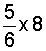Solution:Answer: It will take 6 and 2/3 yards of fabric to make 8 dresses.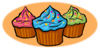Example 2: Renee had a box of cupcakes, of which she gave 1/2 to her friend Juan. Juan gave 3/4 of his share to his friend Elena. What fractional part of the original box of cupcakes did Elena get?

Analysis: To solve this problem, we will multiply these two fractions.

Solution: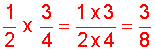Answer: Elena got 3/8 of the original box of cupcakes.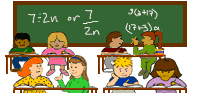Example 3: Nina's math classroom is 6 and 4/5 meters long and 1 and 3/8 meters wide. What is the area of the classroom?

Analysis: To solve this problem, we will multiply these mixed numbers. But first we must convert each mixed number to an improper fraction.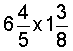Solution:Answer: The area of the classroom is 9 and 7/20 square meters.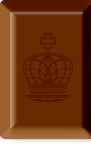Example 4: A chocolate bar is 3/4 of an inch long. If it is divided into pieces that are 3/8 of an inch long, then how many pieces is that?

Analysis: To solve this problem, we will divide the first fraction by the second.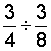Solution: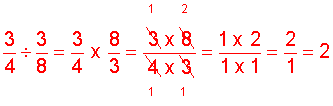Example 5: An electrician has a piece of wire that is 4 and 3/8 centimeters long. She divides the wire into pieces that are 1 and 2/3 centimeters long. How many pieces does she have?

Analysis: To solve this problem, we will divide the first mixed number by the second.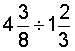Solution:Answer: The electrician has 2 and 5/8 pieces of wire.Example 6: A warehouse has 1 and 3/10 meters of tape. If they divide the tape onto pieces that are 5/8 meters long, then how many pieces will they have?

Analysis: To solve this problem, we will divide the first mixed number by the second. First, we will convert each mixed number into an improper fraction.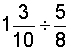Solution: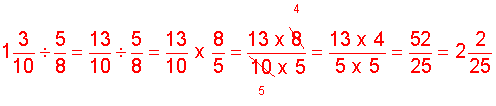Answer: The warehouse will have 2 and 2/25 pieces of tape.

Summary: In this lesson we learned how to solve word problems involving multiplication and division of fractions and mixed numbers.

### Exercises

Directions: Subtract the mixed numbers in each exercise below. Be sure to simplify your result, if necessary. Click once in an ANSWER BOX and type in your answer; then click ENTER. After you click ENTER, a message will appear in the RESULTS BOX to indicate whether your answer is correct or incorrect. To start over, click CLEAR.

Note: To write the mixed number four and two-thirds, enter 4, a space, and then 2/3 into the form.

 1 One batch of cookies contains 1 and 3/4 cups of melted chocolate. How many cups of melted chocolate are needed to make 8 batches of cookies?   ANSWER BOX:  cups   RESULTS BOX:
 2 Todd drank 5/8 of a 24-ounce can of juice. Lila drank 1/3 as much juice as Todd did. How many ounces did Lila drink?   ANSWER BOX:   oz.  RESULTS BOX:
 3 A rectangular area rug has a length of 3 and 2/3 feet and a width of 2 and 3/4 feet. What is the area of the rug?   ANSWER BOX:   sq ft   RESULTS BOX:
 4 Janet has 5 and 3/4 centimeters of licorice. She divides the licorice into pieces that are 1 and 7/8 centimeters long. How many pieces of licorice will she have?   ANSWER BOX:   pieces   RESULTS BOX:
 5 A piece of wood is 15 feet long. How many 3/4-foot sections can be cut from it?   ANSWER BOX:   sections    RESULTS BOX:

 Lessons on Multiplying and Dividing Fractions and Mixed Numbers 1. Multiplying Fractions 2. Multiplying Fractions by Cancelling Common Factors 3. Multiplying Mixed Numbers 4. Reciprocals 5. Dividing Fractions 6. Dividing Mixed Numbers 6. Solving Word Problems 7. Practice Exercises 8. Challenge Exercises 9. Solutions